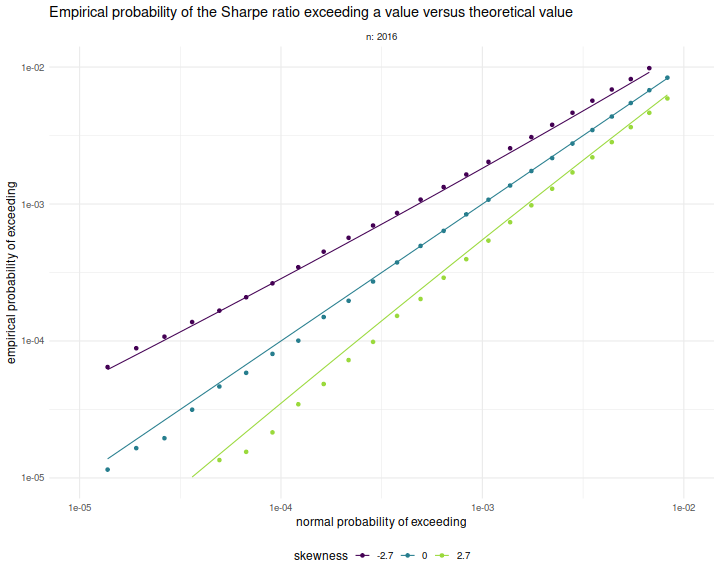# Sharpe Ratio

## Achieved Signal Noise Ratio via Cross Validation

Some of the research on the problem of "overfitting" of quantitative strategies (including my own) might be better described as research on "over-optimism". That is because the analysis tends to view the strategies as something one stumbles upon, without any in-sample tinkering. If that is the case, the Sharpe ratio is nearly unbiased for the signal-noise ratio, and the only statistical sin is selecting the best strategy among many without some kind of multiple hypothesis test correction. However, strategies tend to be generated based on some in-sample overfitting beyond just selection. As an example, suppose you observed $$n$$ days of returns on $$k$$ assets in your universe, then constructed the Markowitz portfolio based on that data. If your strategy is to hold that Markowitz portfolio, it is a bit trickier to de-bias the in-sample Sharpe ratio.

For the specific problem of estimating what I call the achieved signal-noise ratio of the Markowitz portfolio, one can use the Sharpe Ratio Information Criterion of Paulsen and Söhl. However, I suspect most practicing quants would fall back to cross validation. Cross validation is a folk remedy prescribed to cure all ills, probably beneficial but of unknown efficacy. In the usual $$m$$ fold cross validation, one splits the data into $$m$$ equally sized pieces, fits the Markowitz portfolio on all but one of those $$m$$ pieces, then simulates returns on that piece. This is repeated holding out each of the $$m$$ validation sets. The result is a simulated time series over the $$n$$ days of data, which one then computes the Sharpe ratio on. I commonly used a fancier version of cross validation, called walk forward, where one only estimated the portfolio on data prior to the validation set, which results in a slightly truncated resultant time series. The $$m$$-fold and walk-forward cross validation techniques are illustrated below for the case of $$m=5$$ folds.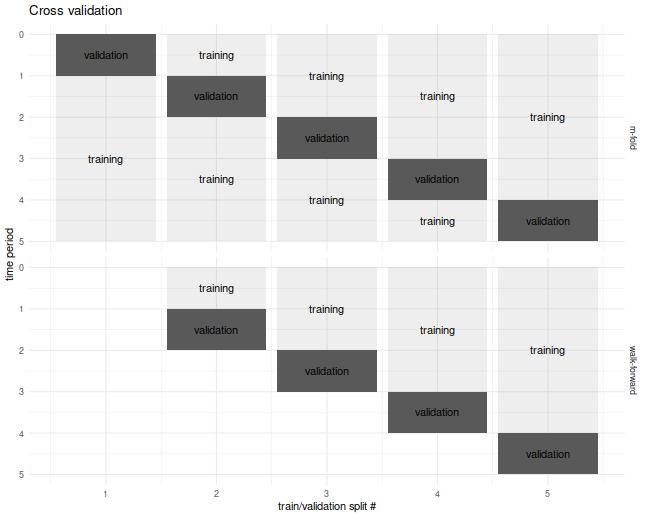## Cross Validation is Broken?

Although I used cross-validation in this way for years, I never tested it until now. It seemed "obvious" that it would provide unbiased results. It turns out I was wrong, as simple simulations will show.

In our set up, cross validation is designed to estimate the achieved signal-noise ratio. "Achieved" means we are considering the signal-noise ratio of the sample Markowitz portfolio. This is a quantity that is both random (depends on the sample) and unobservable (depends on the population mean and covariance). In the simulations below I perform $$10$$-fold regular and walk-forward cross validation, constructing the Markowitz portfolio on training data, then generating a single time series of returns, computing the Sharpe on those returns. I also compute the achieved signal-noise ratio of the Markowitz portfolio on the whole sample. I vary the maximal signal-noise ratio of the population, as well as the number of assets. For every setting of the population parameters I perform 50000 simulations, then average the cross validated Sharpes and the achieved signal-noise ratios over the simulations.

Below I plot the bias of the Sharpes estimated via regular and walk-forward cross validation, defined as the Sharpe minus the achieved signal-noise ratio. We see that, in annual terms, both cross validation techniques are severely biased, and walk-forward gets worse as the maximal signal-noise ratio increases. The bias is negative, which is to say we _under_estimate the achieved signal-noise ratio.

suppressMessages({
library(dplyr)
library(tidyr)
library(tibble)
library(future.apply)
})

ope <- 252
# compute markowitz portfolio
comp_mp <- function(X) { solve(cov(X),colMeans(X)) }
# compute sr
comp_sr <- function(x,na.rm=TRUE) { mean(x,na.rm=na.rm) / sd(x,na.rm=na.rm) }
# run simulations
simit <- function(n,k,zeta,nsim=1000,nfolds=10) {
require(future.apply)
won <- seq(n)
cvidx <- (won %% nfolds) + 1
mu <- zeta / sqrt(k)
vals <- future_replicate(nsim,{
X <- matrix(rnorm(n*k,mean=mu),ncol=k)
kfret <- rep(0,n)
wfret <- rep(NA_real_,n)
for (folnum in unique(cvidx)) {
oosi <- cvidx==folnum
oos <- X[oosi,]
iis <- X[!oosi,]
mp <- comp_mp(iis)
kfret[oosi] <- oos %*% mp
# walk forward
iisi <- cvidx < folnum
if (any(iisi)) {
iis <- X[iisi,]
mp <- comp_mp(iis)
wfret[oosi] <- oos %*% mp
}
}
cv_sr <- comp_sr(kfret)
wf_sr <- comp_sr(wfret,na.rm=TRUE)
achieved_snr <- sum(mu * mp) / sqrt(sum(mp^2))
c(cv_sr,wf_sr,achieved_snr,sqrt(ope)*mean(kfret),sd(kfret))
})
setNames(data.frame(sqrt(ope) * t(vals)),c('CV','walkforward','achieved','kf_mean','kf_sd'))
}

nday <- ope*5
nsim <- 50000

params <- crossing(tibble(zeta=seq(0.125,1.875,length.out=7)/sqrt(ope)),tibble(k=c(5,10)))

set.seed(1234)
plan(multiprocess)
resu <- params %>%
group_by(zeta,k) %>%
summarize(foo=list(simit(n=nday,k=k,zeta=zeta,nsim=nsim))) %>%
ungroup() %>%
unnest(foo)
plan(sequential)

# aggregate the results
mresu <- resu %>%
tidyr::gather(key=series,value=value,-zeta,-k,-achieved) %>%
mutate(bias=value - achieved) %>%
group_by(zeta,k,series) %>%
summarize(emp_bias=mean(bias),
emp_sbias=sd(bias),
count=n()) %>%
ungroup() %>%
mutate(emp_biase=emp_sbias / sqrt(count))

ph <- mresu %>%
dplyr::filter(series %in% c('CV','walkforward')) %>%
mutate(showser=case_when(series=='CV'  ~ 'cross val. SR',
series=='walkforward' ~ 'w.f. cross val. SR',
TRUE ~ 'error')) %>%
ggplot(aes(sqrt(ope)*zeta,emp_bias,
linetype=showser,
group=interaction(k,showser))) +
geom_hline(yintercept=0,linetype=3,alpha=0.8) +
geom_point(aes(shape=showser),alpha=0.9) +
geom_errorbar(aes(ymin=emp_bias - emp_biase,ymax=emp_bias + emp_biase),width=0.1) +
geom_line(alpha=0.5) +
facet_wrap(~k,labeller=label_both) +
labs(x=expression(zeta['*']~~(yr^{-1/2})),
y=expression(bias~~(yr^{-1/2})),
shape='series',
linetype='series')
print(ph)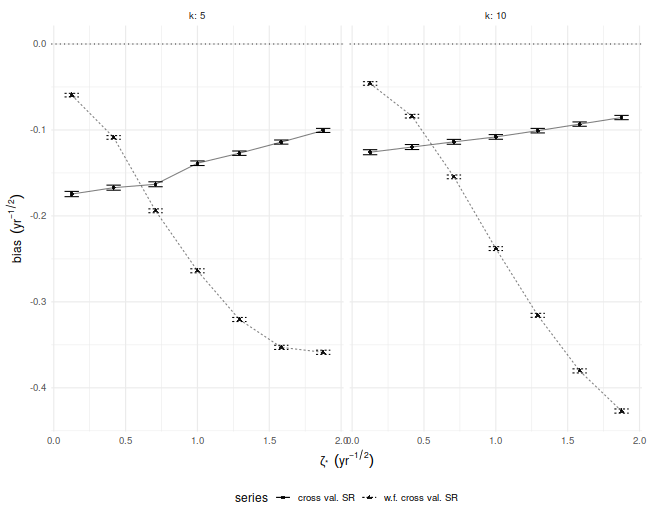## Where is the bias?

Despite the relative simplicity of the simulations, I was convinced they contained a bug. How could cross-validation be so broken? I tried increasing the number of folds, which only made the problem worse! In my debugging I noticed that the estimate of the mean return of the Markowitz portfolio seemed unbiased. How can one have an unbiased estimate of the mean, but a biased estimate of the Sharpe ratio? The answer to that question is that the sample mean and sample standard deviation are not independent under cross validation.

We illustrate that here by performing simulations where the population mean is the zero vector. For each simulation we compute the numerator and denominator of the cross-validated Sharpe ratio, namely the mean and standard deviation of the simulated returns on validation sets. We then scatter the means versus the standard deviations. There is a clear correlation here. I believe this is a well-known effect in cross-validation. Moreover, if your standard deviation is positively correlated with your mean, it will clearly bias your Sharpe ratio. This is simple to understand intuitively, we leave it as an exercise to show how that bias is a function of the correlation.

nday <- ope*5
nsim <- 10000

set.seed(1234)
plan(multiprocess)
atz <- simit(n=nday,k=5,zeta=0,nsim=nsim)
plan(sequential)

# scatter em
ph <- atz %>%
ggplot(aes(kf_sd,kf_mean)) +
geom_point(alpha=0.02) +
stat_smooth() +
geom_hline(yintercept=0,linetype=3) +
geom_rug(alpha=0.02,sides='rb') +
labs(x=expression(sd~of~rets~~(yr^{-1/2})),
y=expression(mean~of~rets~~(yr^{-1})))
print(ph)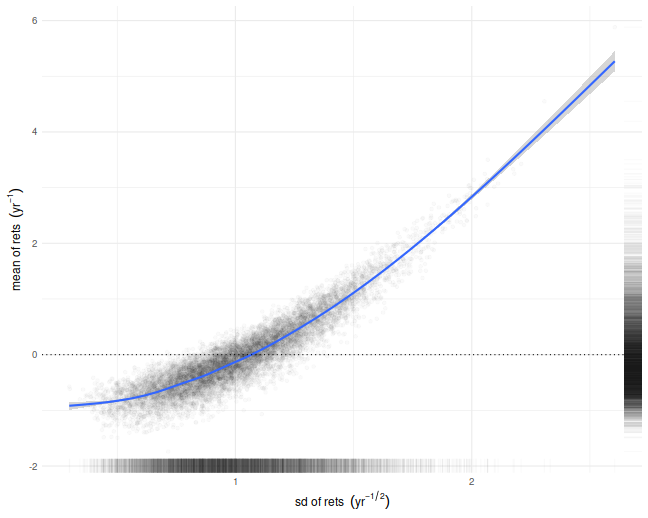## What can be done?

If you intended to trade the Markowitz portfolio, you could probably debias the cross-validated estimates by some mathematical wizardry. However, most quants deploy more complicated trading strategies, which would be harder to analyze. My only suggestion at the moment is to instead use an average of Sharpes approach: perform cross-validation as usual, but instead of computing a single time series of returns, compute the Sharpe ratio on each validation set, then average them. In a future blog post I will show that this has far less bias for our toy problem.

## A Sign Sharpe

Consider the following challenge: estimate the Signal-Noise ratio of an asset given a single observation of returns of that asset, $$x$$. The task seems impossible: you can easily estimate the mean, but how are you going to estimate the standard deviation with just one observation? Consider, however, that for most assets the expected value of returns is very small compared to the volatility, so if you wanted to estimate the variance of returns, $$x^2$$ would be a good guess. Thus $$\left|x\right|$$ would be a passable estimate for the standard deviation. Given that $$x$$ is an unbiased estimate of the mean, then

$$\frac{x}{\left|x\right|} = \operatorname{sign}(x)$$

is our candidate estimator of the Signal-Noise ratio.

It turns out this will work, up to scaling. Suppose that returns are Gaussian. Then

$$E\left[ \operatorname{sign}(x) \right] = 2\Phi\left(\frac{\mu}{\sigma}\right) - 1 \approx \sqrt{\frac{2}{\pi}} \frac{\mu}{\sigma}.$$

Thus $$\sqrt{\frac{\pi}{2}} \operatorname{sign}(x)$$ is a nearly unbiased estimator of the Signal-Noise ratio.

Since this estimator has only two possible values, it is going to be very noisy. However, if you had $$n$$ observations $$x_t$$, you could average them together as

$$\check{\zeta} = \frac{1}{n}\sqrt{\frac{\pi}{2}} \sum_{1 \le t \le n}\operatorname{sign}\left(x_t\right).$$

Call this the sign Sharpe ratio. Note that it is just a rescaled version of the cringe-worthy "winning days percentage" statistic some portfolio managers advertise.

There are two obvious problems with the sign Sharpe ratio.

1. It is easy to show that the standard error of $$\check{\zeta}$$ is $$\sqrt{\frac{\pi}{2n}}$$, which is about 20% bigger than that of the moment-based Sharpe ratio.
2. It is only nearly unbiased for Gaussian returns, and can have serious bias for skewed returns.

The latter point is a serious problem. One can easily construct returns streams with absurdly high or low values of winning days percentage, and somewhat arbitrary signal-noise ratio. By using the Edgeworth expansion, one can show that the expected value of $$\check{\zeta}$$ is approximately

$$E\left[ \check{\zeta} \right] \approx \zeta - \frac{\gamma_1}{6},$$

where $$\zeta=\mu/\sigma$$ is the Signal-Noise ratio, and $$\gamma_1$$ is the standardized skewness of returns. Of course, if you somehow knew the skew of returns, you could correct for them. More interesting would be to find a way to estimate $$\gamma_1$$ from a single observation, though I would argue that $$c\operatorname{sign}\left(x\right)$$ is probably the best candidate.

Let's confirm these findings experimentally. Consider the case where $$x_t$$ are drawn from a Beta distribution, shifted to have a fixed mean. We fix the Signal-Noise ratio to be 0.1, and tweak the two shape parameters of the Beta distribution, $$\alpha$$ and $$\beta$$, to vary the skewness of returns. We plot the empirical mean value of $$\check{\zeta}$$ for $$n=10^7$$, along with the approximate theoretical value, versus the skewness. We see very good agreement between the two except for the case where $$\alpha$$ and $$\beta$$ are small, where the higher order moments which we have discarded are no longer negligible. We would note that this is also likely to be a problem for real world asset returns.

library(dplyr)
library(tidyr)
library(tibble)
library(ggplot2)

# generate shifted beta variates with a fixed SNR zeta
genb <- function(n,zeta,alpha,beta) {
mu <- alpha / (alpha+beta)
sg <- sqrt(alpha*beta/((alpha+beta+1)*(alpha+beta)^2))
x <- (sg*zeta - mu) + rbeta(n,shape1=alpha,shape2=beta)
}
signsr <- function(x) { sqrt(pi/2) * mean(sign(x)) }

zeta <- 0.1
set.seed(12345)
sims <- crossing(tibble(alpha=c(5,50,500)),tibble(beta=c(10,100,1000))) %>%
group_by(alpha,beta) %>%
summarize(simzet=signsr(genb(n=10000000,zeta=zeta,alpha=alpha,beta=beta)),
skew=2*(beta-alpha)*sqrt(beta+alpha+1)/((alpha+beta+2)*sqrt(alpha*beta))) %>%
ungroup() %>%
mutate(esign=zeta - skew / 6)

# plot them
sims %>%
ggplot(aes(skew,simzet)) +
geom_point(aes(color=factor(alpha),shape=factor(beta))) +
geom_line(aes(y=esign),linetype=3,alpha=0.5) +
labs(x=expression(gamma),
y='mean of sign SR',
title='Empirical and approximate theoretical mean value of sign SR',
color=expression(alpha),shape=expression(beta))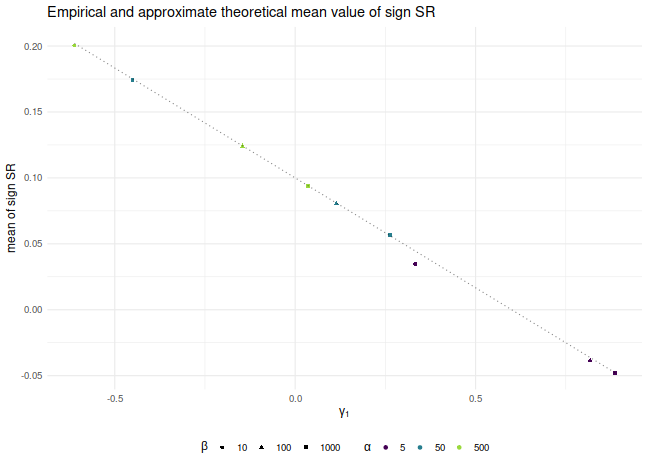## Is it useful?

The sign Sharpe ratio does not seem useful for estimating the Signal-Noise ratio: it is too noisy, and is confounded by higher order moments. However, the challenge of estimating the Sharpe from individual observations was motivated by difficulties bounding the out-of-sample Sharpe ratio from the-in-sample Sharpe ratio using something like VC dimension or Rademacher complexity. The problem is that the standard deviation in the Sharpe computation depends on all the observations. As a side effect, if you increase a single day's returns, it can cause the Sharpe to either increase or decrease. Because of this dependence on the whole sample, the Sharpe ratio can not easily be analyzed by these complexity measures.

## A Sharper Sharpe, Again

Previously on this blog we had performed a fair amount of testing of the "drawdown-based estimator" of the signal-noise ratio, as proposed by Damien Challet. All that analysis was based on the 1.1 version of the sharpeRratio package, written by Challet himself. There was a bug (or bugs) in that package that caused the estimator to be biased, which could also appear as improved efficiency over the traditional "moment-based" estimator due to Sharpe (or Gosset, rather) via shrinkage to zero. Here we analyze the 1.2 version of the package, which presumably fixes this issue.

## Checking for bias

Here I perform some simulations to check for bias of the estimator. I draw 128 days of daily returns from a $$t$$ distribution with $$\nu=4$$ degrees of freedom. I then compute: the moment-based Sharpe ratio; the moment-based Sharpe ratio, but debiased using higher order moments; the drawdown estimator from the 1.2 version of the package; the drawdown estimator from the 1.2 version of the package, but feeding $$\nu$$ to the estimator. I do this for 10,000 draws of returns. I repeat for 256 days of data, and for the population Signal-Noise ratio varying from 0.30 to 1.5 in "annualized units" (per square root year), assuming 252 trading days per year. I use doFuture to run the simulations in parallel.

suppressMessages({
library(dplyr)
library(tidyr)
library(tibble)
library(SharpeR)
library(sharpeRratio)
# https://cran.r-project.org/web/packages/doFuture/vignettes/doFuture.html
library(doFuture)
registerDoFuture()
plan(multiprocess)
})
# only works for scalar pzeta:
onesim <- function(nday,pzeta=0.1,nu=4) {
x <- pzeta + sqrt(1 - (2/nu)) * rt(nday,df=nu)
srv <- SharpeR::as.sr(x,higher_order=TRUE)
# mental note: this is much more awkward than it should be,
# let's make it easier in SharpeR!
#ssr <- mean(x) / sd(x)
# moment based:
ssr <- srv$sr # debiased ssr_b <- ssr - SharpeR::sr_bias(snr=ssr,n=nday,cumulants=srv$cumulants)

sim <- sharpeRratio::estimateSNR(x)
# this cheats and gives the true nu to the estimator
cht <- sharpeRratio::estimateSNR(x,nu=nu)
c(ssr,ssr_b,sim$SNR,cht$SNR)
}
repsim <- function(nrep,nday,pzeta=0.1,nu=4) {
dummy <- invisible(capture.output(jumble <- replicate(nrep,onesim(nday=nday,pzeta=pzeta,nu=nu)),file='/dev/null'))
retv <- t(jumble)
colnames(retv) <- c('sr','sr_unbiased','ddown','ddown_cheat')
invisible(as.data.frame(retv))
}
manysim <- function(nrep,nday,pzeta,nu=4,nnodes=5) {
if (nrep > 2*nnodes) {
# do in parallel.
nper <- table(1 + ((0:(nrep-1) %% nnodes)))
retv <- foreach(i=1:nnodes,.export = c('nday','pzeta','nu')) %dopar% {
repsim(nrep=nper[i],nday=nday,pzeta=pzeta,nu=nu)
} %>%
bind_rows()
} else {
retv <- repsim(nrep=nrep,nday=nday,pzeta=pzeta,nu=nu)
}
retv
}
# summarizing function
sim_summary <- function(retv) {
retv %>%
tidyr::gather(key=metric,value=value,-pzeta,-nday) %>%
filter(!is.na(value)) %>%
group_by(pzeta,nday,metric) %>%
summarize(meanvalue=mean(value),
serr=sd(value) / sqrt(n()),
rmse=sqrt(mean((pzeta - value)^2)),
nsims=n()) %>%
ungroup() %>%
arrange(pzeta,nday,metric)
}

ope <- 252
pzeta <- seq(0.30,1.5,by=0.30) / sqrt(ope)

params <- tidyr::crossing(tibble::tribble(~nday,128,256),
tibble::tibble(pzeta=pzeta))

nrep <- 10000
set.seed(1234)
system.time({
results <- params %>%
group_by(nday,pzeta) %>%
summarize(sims=list(manysim(nrep=nrep,nnodes=7,pzeta=pzeta,nday=nday))) %>%
ungroup() %>%
tidyr::unnest(cols=c(sims))
})

   user  system elapsed
9439.36    7.13 1429.51


I compute the mean of each estimator over the 10,000 draws, divide that mean estimate by the true Signal-Noise Ratio, then plot versus the annualized SNR. I plot errobars at plus and minus one standard error around the mean. The ratio should be one, any deviation from which is geometric bias in the estimator. Previously this plot showed the drawdown estimator consistently estimating a value around 70% of the true value, a problem which seems to have been fixed, as it now shows values around 95% of the true value. The moment estimator shows a slight positive bias, which is decreasing in sample size, as described by Bao and Miller and Gehr. The higher order moment correction mitigates this effect somewhat for the moment estimator.

library(ggplot2)
ph <- results %>%
sim_summary() %>%
mutate(metric=case_when(.$metric=='ddown' ~ 'drawdown estimator v1.1', .$metric=='ddown_two' ~ 'drawdown estimator v1.2',
.$metric=='ddown_cheat' ~ 'drawdown estimator v1.2, nu given', .$metric=='sr_unbiased' ~ 'moment estimator, debiased',
.$metric=='sr' ~ 'moment estimator (SR)', TRUE ~ 'error')) %>% mutate(bias = meanvalue / pzeta, zeta_pa=sqrt(ope) * pzeta, serr = serr) %>% ggplot(aes(zeta_pa,bias,color=metric,ymin=bias-serr,ymax=bias+serr)) + geom_line() + geom_point() + geom_errorbar(alpha=0.5) + geom_hline(yintercept=1,linetype=2,alpha=0.5) + facet_wrap(~nday,labeller=label_both) + scale_y_log10() + labs(x='Signal-noise ratio (per square root year)', y='empirical expected value of estimator, divided by actual value', color='estimator', title='geometric bias of SR estimators') print(ph)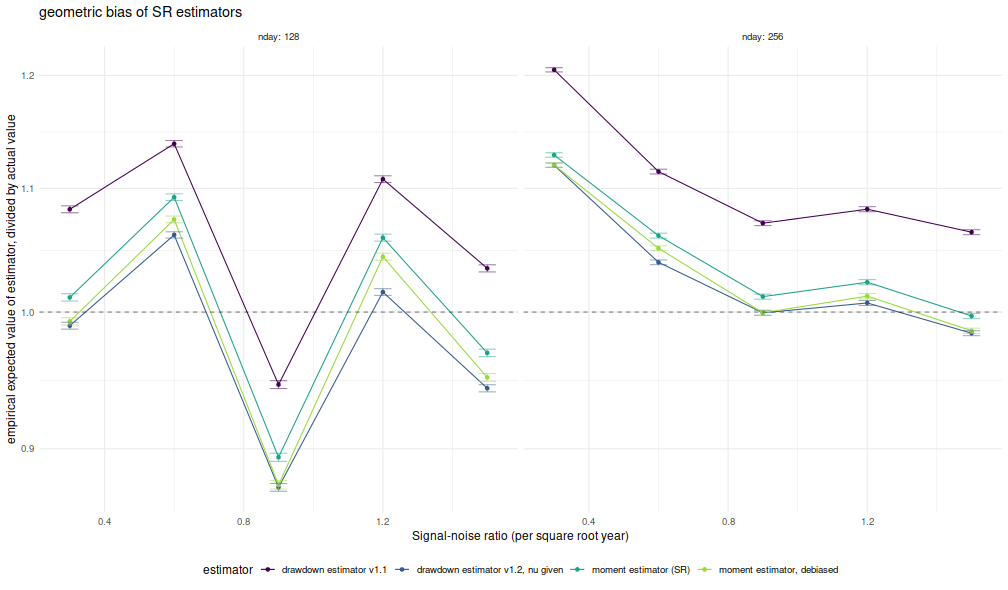I now plot the 'relative efficiency' as in Figure 4 of version 6 of Challet's paper. This is the ratio of the mean square error of the moment-estimator to the mean square error of the drawdown-estimator, again as a function of the true (annualized) signal-noise ratio, with different lines for the number of days simulated. Challet's plot shows this line as approximately 5, while we see values of around 1.25 or so. That is, we see only modest improvements in efficiency for the drawdown estimator, and not the putative huge gains in efficiency in the paper. library(ggplot2) ph <- results %>% sim_summary() %>% filter(metric %in% c('sr','ddown')) %>% select(-meanvalue,-serr,-nsims) %>% tidyr::spread(key=metric,value=rmse) %>% mutate(eff=(sr/ddown)^2) %>% mutate(zeta_pa=sqrt(ope) * pzeta) %>% ggplot(aes(zeta_pa,eff,color=factor(nday))) + geom_line() + geom_point() + geom_hline(yintercept=1,linetype=3) + labs(x='Signal-noise ratio (per square root year)', y='relative efficiency of drawdown to moment estimator', color='num days', title='Efficiency ofSR estimators') print(ph)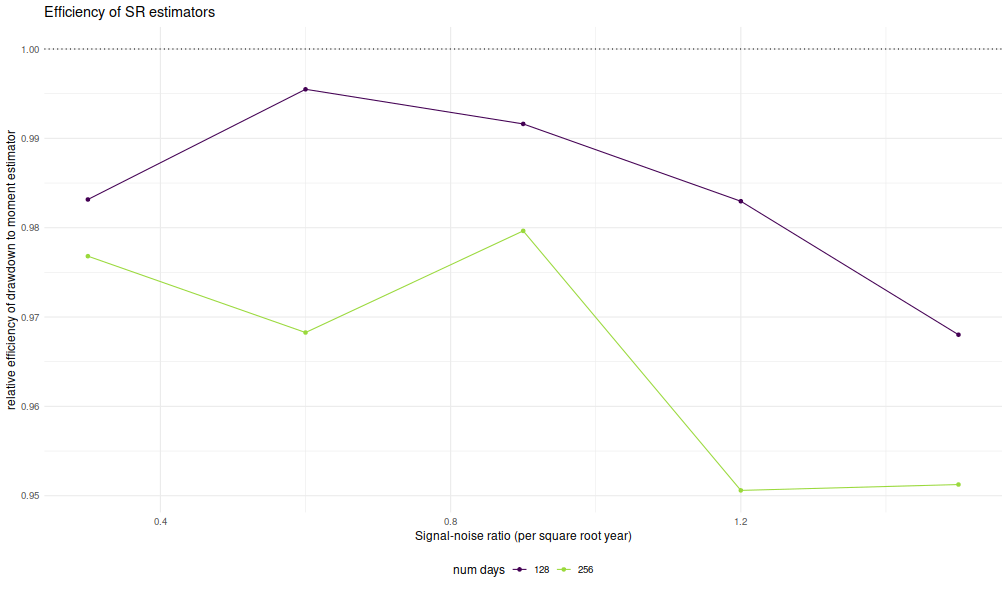Thus it appears that the 1.2 version of the package fixes the bias issues in the initial release. Click to read and post comments #### Jan 02, 2020 ## Probability of large deviation of the Sharpe ratio In chapter 4 of our Short Sharpe Course, we analyzed in great detail the standard error of the Sharpe ratio under a number of deviations from the assumptions of i.i.d. normal returns. By showing that the standard error does not differ too much from the nominal value, we established that hypothesis testing with moderate type I error rates is largely achievable. However, these results do not necessarily support testing with very small type I rates, as the tail distribution of the Sharpe ratio may be far from Gaussian. It turns out there are known bounds on large deviations of the $$t$$-statistic which we can directly translate into equivalent facts regarding the Sharpe. It is not surprising that one of these results was coauthored by Peter Hall, who wrote a book on the convergence rates of the Central Limit Theorem. Under the null hypothesis, $$\zeta=0$$, Wang and Hall showed that $$\mathcal{P}\left(\zeta \ge q\right) \approx \left(1 - \Phi\left(\frac{n}{n-1}\sqrt{n}q\right)\right) \operatorname{exp}\left(-\frac{1}{3} \left(\frac{nq}{n-1}\right)^3 n \gamma_1 \right).$$ Here $$\gamma_1$$ is the skewness of returns and $$\Phi\left(x\right)$$ is the Gaussian distribution, and thus the approximation (which holds up to a factor in $$n^{-1}$$) compares the exceedance probability of the Sharpe ratio to the equivalent Gaussian law. For moderately skewed returns and modestly sized $$n$$, we expect the correction factor to be around $$1\pm 0.1$$ or so. This means that the type I rate assuming a normal distribution for the Sharpe is usually'' within 10% of nominal. It is worth noting that the deviance from the normal approximation is affected not by kurtosis per se, but by the skewness, which is to be expected from the Berry-Esseen theorem. We note that in the case $$\zeta\ne0$$, a more complicated version of the approximation holds, but we defer this to our updated Short Sharpe Course. ## Simulations Here we confirm the relationship above empirically. We draw returns from a Lambert W $$\times$$ Gaussian'' distribution, with the skew parameter, $$\delta$$ varying from $$-0.4$$ to $$0.4$$, and we set $$n$$ to 8 years of daily data. For each setting of the skew we perform many simulations under the null hypothesis, $$\zeta=0$$, then compute the empirical probability that the Sharpe ratio exceeds some value $$q$$. suppressMessages({ library(dplyr) library(tidyr) library(magrittr) library(future.apply) library(LambertW) library(tibble) }) #Lambert x Gaussian gen_lambert_w <- function(n,dl = 0.1,mu = 0,sg = 1) { require(LambertW,quietly=TRUE) suppressWarnings({ Gauss_input = create_LambertW_input("normal", beta=c(0,1)) params = list(delta = c(0), gamma=c(dl), alpha = 1) LW.Gauss = create_LambertW_output(Gauss_input, theta = params) #get the moments of this distribution moms <- mLambertW(beta=c(0,1),distname=c("normal"),delta = 0,gamma = dl, alpha = 1) }) if (!is.null(LW.Gauss$r)) {
# API changed in 0.5:
samp <- LW.Gauss$r(n=n) } else { samp <- LW.Gauss$rY(params)(n=n)
}
samp <- mu  + (sg/moms$sd) * (samp - moms$mean)
}
moms_lambert_w <- function(dl = 0.1,mu = 0,sg = 1) {
require(LambertW,quietly=TRUE)
suppressWarnings({
Gauss_input = create_LambertW_input("normal", beta=c(0,1))
params = list(delta = c(0), gamma=c(dl), alpha = 1)
LW.Gauss = create_LambertW_output(Gauss_input, theta = params)
#get the moments of this distribution
moms <- mLambertW(beta=c(0,1),distname=c("normal"),delta = 0,gamma = dl, alpha = 1)
})
moms$mean <- mu moms$sd <- sg
return(moms)
}

# columnwise Sharpe
colsr <- function(X) { (colMeans(X) / apply(X,2,sd)) }
srsims <- function(nsim,nday,...) { colsr(matrix(gen_lambert_w(nsim*nday,...),nrow=nday)) }
manysims <- function(nsim,nday,dl=0.1,cuts=100) {
require(future.apply)
as.numeric(future_replicate(cuts,{ srsims(ceiling(nsim/cuts),nday=nday,dl=dl) }))
}
propex <- function(srs,vals=seq(0,0.5,length.out=301)) {
require(zipper)  # install.github('shabbychef/zipper')
places <- zipper::zip_le(sort(srs),vals)
1 - (places + 0.5) / (length(srs) + 1)
}
exceedance <- function(nday,dl=0.1,nsim=1e4,vals=seq(0,0.5,length.out=301)) {
srs <- manysims(nsim=nsim,nday=nday,dl=dl)
ppp <- propex(srs=srs,vals=vals)
moms <- moms_lambert_w(dl=dl)
tibble(vals=vals,prop=ppp,skewness=moms\$skewness)
}

params <- tidyr::crossing(tibble::tribble(~n,8*252),
tibble::tribble(~dl,-0.4,0,0.4))

# sims:
nsim <- 1e6
library(future.apply)
plan(multiprocess,workers=7)
set.seed(1234)
suppressMessages({
resu <- params %>%
group_by(n,dl) %>%
summarize(sims=list(exceedance(nday=n,dl=dl,nsim=nsim))) %>%
ungroup() %>%
tidyr::unnest(cols=c(sims))
})
plan(sequential)


Here we plot the empirical exceedance probabilities versus $$1 - \Phi\left(\frac{n}{n-1}\sqrt{n}q\right)$$, with lines for the right hand side of the approximation above. We see that the approximation matches the experiments fairly well.

library(ggplot2)
ph <- resu %>%
mutate(norm_law=pnorm(sqrt(n)*(n/(n-1))*vals,lower.tail=FALSE)) %>%
mutate(hall_law=norm_law * exp(-(1/3)*((n*vals/(n-1))^3) * n * skewness)) %>%
mutate(fskew=factor(signif(skewness,2))) %>%
ggplot(aes(norm_law,prop,color=fskew,group=interaction(n,dl))) +
geom_point() +
geom_line(aes(y=hall_law))+
scale_x_log10(limits=c(1e-5,0.01)) +
scale_y_log10(limits=c(1e-5,0.01)) +
facet_wrap(~n,labeller=label_both) +
labs(x='normal probaility of exceeding',
y='empirical probability of exceeding',
color='skewness',
title='Empirical probability of the Sharpe ratio exceeding a value versus theoretical value')
print(ph)